• 二维随机变量的联合分布函数：二维随机变量的联合分布函数二维离散型随机变量概率分布：二维随机变量的概率分布二维离散型随机变量的边缘分布：二维离散型随机变量的边缘分布二维离散型随机变量的条件分布：二维...
二维随机变量的联合分布函数：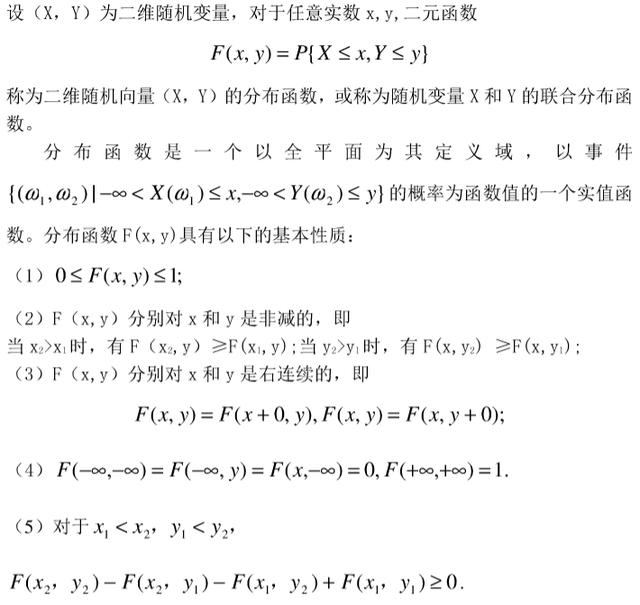二维随机变量的联合分布函数二维离散型随机变量的概率分布：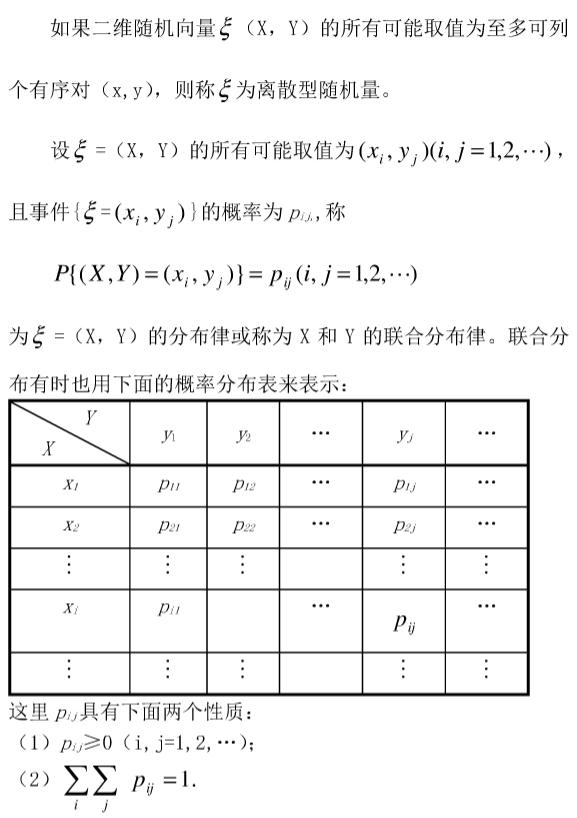二维随机变量的概率分布二维离散型随机变量的边缘分布：二维离散型随机变量的边缘分布二维离散型随机变量的条件分布：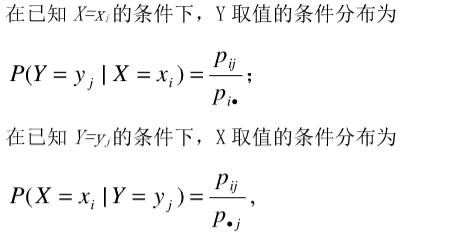二维离散型随机变量的条件分布题型一：求二维离散随机变量的联合分布律和条件概率分布例1：设随机变量X在1，2，3三个数字中等可能的取值，随机变量Y在1~X中等可能的取一整数值，试求二维随机变量(X,Y)的概率分布。解：由乘法公式，可得P{X=1,Y=1}=P{X=1}P{Y=1|X=1}=1/3*1=1/3;P{X=2,Y=1}=P{X=2}P{Y=1|X=2}=1/3*(1/2)=1/6;P{X=2,Y=2}=P{X=1}P{Y=2|X=2}=1/3*(1/2)=1/6;P{X=3,Y=1}=P{X=3}P{Y=1|X=3}=1/3*(1/3)=1/9;P{X=3,Y=2}=P{X=3}P{Y=2|X=3}=1/3*(1/3)=1/9;P{X=3,Y=3}=P{X=3}P{Y=3|X=3}=1/3*(1/3)=1/9;P{X=1,Y=2}=P{X=1,Y=3}=P{X=2,Y=3}=0.所以，(X,Y)的概率分布为：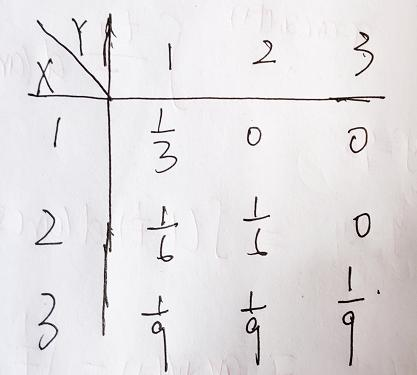进一步得到边缘分布：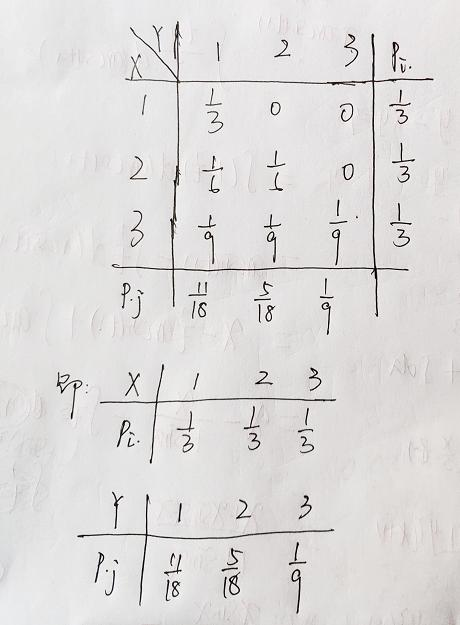边缘分布的求法求在Y=2条件下X的条件分布：由上面解答可知P{Y=2}=5/18,因此P{X=1|Y=2}=P{X=1,Y=2}/P{Y=2}=0;P{X=2|Y=2}=P{X=2,Y=2}/P{Y=2}=(1/6)/(5/18)=3/5;P{X=3|Y=2}=P{X=3,Y=2}/P{Y=2}=(1/9)/(5/18)=2/5;得到在Y=2的条件下随机变量X的条件分布为：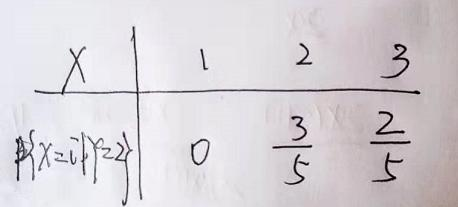展开全文• 使用多种方法实现二维离散随机变量概率分布的计算. 可以用于计算互信息等.
一. 定义

Joint probability distribution:

给定至少两个随机变量X,Y,…, 它们的联合概率分布(Joint probability distribution)指的是每一个随机变量的值落入特定范围或者离散点集合内的概率. 对于只有两个随机变量的情况, 称为二元分布(bivariate distribution).

联合概率分布可以使用联合累计分布函数(joint cumulative distribution function), 连续随机变量的联合概率密度函数(joint probability density function)或者离散变量的联合概率质量函数(joint probability mass function)来描述. 由此又衍生出两个概念: 边缘分布(marginal distribution)和条件概率分布(conditional probability distribution).

二. 离散变量的联合概率质量函数公式

公式:是给定X=x<!--//--><![CDATA[//><!--
X=x
//--><!]]>的Y=y<!--//--><![CDATA[//><!--
Y=y
//--><!]]>的条件概率.
而且有:如果X<!--//--><![CDATA[//><!--
X
//--><!]]>和Y<!--//--><![CDATA[//><!--
Y
//--><!]]>相互独立:如果X<!--//--><![CDATA[//><!--
X
//--><!]]>和Y<!--//--><![CDATA[//><!--
Y
//--><!]]>条件不独立(conditionally dependent): P(X=x and Y=y)=P(X=x)⋅P(Y=y|X=x)<!--//--><![CDATA[//><!--
P(X=x \ and\  Y=y)=P(X=x)·P(Y=y|X=x)
//--><!]]>
也可以使用联合累计分布函数的差分来计算: 联合累计分布函数定义是:所以F(x,y)<!--//--><![CDATA[//><!--
F(x,y)
//--><!]]>的导数(差分)就是P(X=x and Y=y)<!--//--><![CDATA[//><!--
P(X=x \ and\  Y=y)
//--><!]]>

三. 使用Matlab计算离散2D联合分布

参考: Calculating a 2D joint probability distribution
离散2D联合分布可用于计算两张图片的互信息MI.

0. 定义两个离散的随机变量.

有N个点分布在边长为1的正方形区域内. 把正方形分为K1*K2的小矩形. 统计每个小矩形内的点的个数.

% Data
N = 1e5;    % number of points
xy = rand(N, 2);    % coordinates of points
xy(randi(2*N, 100, 1)) = 0;    % add some points on one side
xy(randi(2*N, 100, 1)) = 1;    % add some points on the other side
xy(randi(N, 100, 1), :) = 0;    % add some points on one corner
xy(randi(N, 100, 1), :) = 1;    % add some points on one corner
inds= unique(randi(N, 100, 1));
xy(inds, :) = repmat([0 1], numel(inds), 1);    % add some points on one corner
inds= unique(randi(N, 100, 1));
xy(inds, :) = repmat([1 0], numel(inds), 1);    % add some points on one corner

% Intervals for rectangles
K1 = ceil(sqrt(N/5));    % number of intervals along x
K2 = K1;    % number of intervals along y
int_x = [0:(1 / K1):1];    % intervals along x
int_y = [0:(1 / K2):1];    % intervals along y

1. 从定义出发, 使用for循环:

tic
count_cells = zeros(K1, K2);
for k1 = 1:K1
inds1 = (xy(:, 1) >= int_x(k1)) & (xy(:, 1) < int_x(k1 + 1));
for k2 = 1:K2
inds2 = (xy(:, 2) >= int_y(k2)) & (xy(:, 2) < int_y(k2 + 1));
count_cells(k1, k2) = sum(inds1 .* inds2);% 布尔相乘得到交集点的个数
end
end
toc
% Elapsed time is 39.357691 seconds.

可见使用两重循环的计算时间非常长.

2. 使用hist3函数

N=hist3(X,'Edges',edges)是matlab中专门计算二元分布的函数.
edges是包含两个递增array的cell. 第一维分组edge1是edges{1}, 第二维分组edge2是edges{2}.
也就是: edges1(i)<=X(k,1)<edges1(i+1)<!--//--><![CDATA[//><!--
edges{1}(i) <= X(k,1) < edges{1}(i+1)
//--><!]]>edges2(j)<=X(k,2)<edges2(j+1)<!--//--><![CDATA[//><!--
edges{2}(j) <= X(k,2) < edges{2}(j+1)
//--><!]]>
正好落在edges1(i+1)<!--//--><![CDATA[//><!--
edges{1}(i+1)
//--><!]]>或者edges2(j+1)<!--//--><![CDATA[//><!--
edges{2}(j+1)
//--><!]]>上的点的个数放在N的最后一行或者最后一列. hist3不统计edges范围外的部分. N是一个二维矩阵, 统计的落到每个单元格内的点的个数.

tic
count_cells_hist = hist3(xy, 'Edges', {int_x int_y});
% 注意hist3得到的矩阵是K1+1*K2+1的, 所以把最后一行和一列去掉.
% 最后一行或一列表示的是 X(k,1)= edges{1}(end)或者X(k,2) = edges{2}(end)的点数
count_cells_hist(end, :) = []; count_cells_hist(:, end) = [];
toc
all(count_cells(:) == count_cells_hist(:))
% Elapsed time is 0.017995 seconds.

显然比用两重for循环快多了.

3. 使用矩阵二元操作bsxfun

C = bsxfun(fun,A,B)对A和B做逐个元素的二元操作, 操作由函数 fun指定.
返回的C中, 1表示满足条件, 0 表示不满足条件. 可用的fun有:

fun
operation
@plus
Plus
@minus
Minus
@timesArray
multiply
@rdivideRight
array divide
@ldivideLeft
array divide
@power
Array power
@max
Binary maximum
@min
Binary minimum
@rem
Remainder after division
@mod
Modulus after division
@atan2
@atan2d
Four-quadrant inverse tangent; result in degrees
@hypot
Square root of sum of squares
@eq
Equal
@neNot equal

@ltLess than

@le
Less than or equal to
@gt
Greater than
@ge
Greater than or equal to
@andElement-wise
logical AND
@orElement-wise
logical OR
@xorLogical
exclusive OR
使用bsxfun的matlab代码:

%% bsxfun
tic
xcomps = single(bsxfun(@ge,xy(:,1),int_x));% 10000*143矩阵
ycomps = single(bsxfun(@ge,xy(:,2),int_y));% 10000*143矩阵
% 相当于求CDF
count_again = xcomps.' * ycomps; %' 143x143 = 143x1e5 * 1e5x143
% 差分后是142*142
count_again_fix = diff(diff(count_again')');
toc
% Elapsed time is 0.178316 seconds.
all(count_cells_hist(:) == count_again_fix(:))

bsxfun稍逊于hist3, 可以针对没有statistics toolbox的情况下使用.

4. 使用accumarray

A= accumarray(subs,val)使用subs的元素值作为索引. subs和val是一一对应的. 将subs中相同值对应的val值累加. 也就是说, subs中元素的位置决定了val哪些元素相加, subs中元素的值决定了累加值在输出中的位置. 看matlab help中示例:

Example 1
Create a 5-by-1 vector and sum values for repeated 1-D subscripts: val = 101:105; subs = [1; 2; 4; 2; 4]; A = accumarray(subs, val)

A =
101       % A(1) = val(1) = 101
206       % A(2) = val(2)+val(4) = 102+104 = 206
0         % A(3) = 0
208       % A(4) = val(3)+val(5) = 103+105 = 208

subs中元素值必须是正整数值. 所以在表示分组时, 可以把[0,1]区间变为[1,K1]区间. matlab代码:

%%%%% 第五种方法Using accumarray
% Another approach is to use accumarray to make the joint histogram after we bin the data.
% Starting with int_x, int_y, K1, xy, etc.:
tic
% take (0,1) data onto [1 K1], following A.Dondas approach for easy comparison
ii = floor(xy(:,1)*(K1-eps))+1;
ii(ii<1) = 1; ii(ii>K1) = K1;
jj = floor(xy(:,2)*(K1-eps))+1;
jj(jj<1) = 1; jj(jj>K1) = K1;

% create the histogram and normalize
H = accumarray([ii jj],ones(1,size(ii,1)));
PDF = H / size(xy,1); % for probabilities summing to 1
toc
% Elapsed time is 0.006356 seconds.
all(count_cells_hist(:) == count_again_fix(:))

ms级别! 真是快!

5. 使用mex编译

mex混合编程参考: 在Matlab中使用mex函数进行C/C++混合编程

#include "mex.h"
// http://stackoverflow.com/questions/19745917/calculating-a-2d-joint-probability-distribution
void mexFunction(int nlhs, mxArray *plhs[], int nrhs, const mxArray *prhs[])
{
unsigned long int hh, ctrl;       /*  counters                       */
unsigned long int N, m, n;        /*  size of matrices               */
unsigned long int *xy;            /*  data                           */
unsigned long int *count_cells;   /*  joint frequencies              */
/*  matrices needed */
mxArray *count_cellsArray;

/*  Now we need to get the data */
if (nrhs == 3) {
xy = (unsigned long int*) mxGetData(prhs);
N = (unsigned long int) mxGetM(prhs);//取矩阵的行数
m = (unsigned long int) mxGetScalar(prhs);
n = (unsigned long int) mxGetScalar(prhs);
}

/*  Then build the matrices for the output */
count_cellsArray = mxCreateNumericMatrix(m + 1, n + 1, mxUINT32_CLASS, mxREAL);
count_cells = mxGetData(count_cellsArray);
plhs = count_cellsArray;

hh = 0; /* counter for elements of xy */
/* for all points from 1 to N */
for(hh=0; hh<N; hh++) {
ctrl = (m + 1) * xy[N + hh] + xy[hh];
count_cells[ctrl] = count_cells[ctrl] + 1;
}
}

将代码保存为: joint_dist_points_2D.c. 在matlab cmd中运行:

mex joint_dist_points_2D.c

生成joint_dist_points_2D.mexw32文件.
matlab调用代码:

% Use mex function
tic
xy2 = uint32(floor(xy ./ repmat([1 / K1, 1 / K2], N, 1)));
count_cells = joint_dist_points_2D(xy2, uint32(K1), uint32(K2));
toc
% Elapsed time is 0.011696 seconds.

也是非常快的.
展开全文accumarray mex
• 数学期望离散型随机变量的数学期望1. 两点分布2. 分布3. 泊松分布4. 几何分布5. 超几何分布6. 其他连续性随机变量的数学期望1. 均匀分布2. 指数分布3. 正态分布 离散型随机变量的数学期望 1. 两点分布 2. 项...
目录1. 两点分布2. 二项分布/伯努利分布	【X~B(n, p)】3. 泊松分布【ξ ~ P(λ)】4. 几何分布【ξ ~ Ge(p)】5. 超几何分布 【ξ ~ H(n, M , N)】6. 负二项分布
1. 两点分布

定义： 实验的结果只有两种情况，即随机变量只有两个值，则称随机变量ξ服从两点分布。

分布律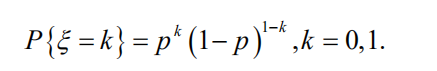-p：表示实验成功的概率。

分布函数

概率密度函数

期望： E(ξ) = p

方差： D(ξ) = p(1-p)

2. 二项分布/伯努利分布	【X~B(n, p)】

定义： n个相互独立的两点分布的组合，具体分布律如下：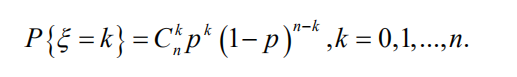（ⅰ）当n为1时，即为两点分布。
（ⅱ）当n趋于无穷大时，即为泊松分布。

分布函数：

概率密度函数：

期望： E(ξ) = np

方差： D(ξ) = np(1-p)

✈二项分布最大值问题。
3. 泊松分布【ξ ~ P(λ)】

定义：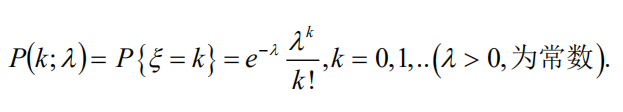
-λ：（一次实验中，实验成功的概率pn ）× （总的试验次数n）

分布函数

概率密度函数

期望： E(ξ) = λ

方差： D(ξ) = λ

泊松定理
4. 几何分布【ξ ~ Ge§】

分布函数
概率密度函数
期望： E(ξ) = 1/p
方差： D(ξ) = (1-p) / p2

几何分布的无记忆性
5. 超几何分布 【ξ ~ H(n, M , N)】

分布函数
概率密度函数
期望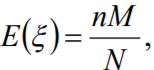方差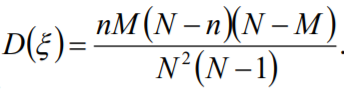超几何分布的二项分布近似：
当n<<N时，

6. 负二项分布
期望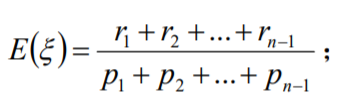方差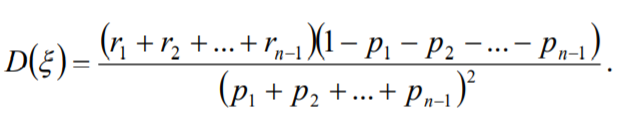展开全文• 文章目录二元随机变量，离散型随机变量分布律二元随机变量二元离散型随机变量（一）离散型随机变量的联合概率分布律联合分布律的性质 二元随机变量，离散型随机变量分布律 二元随机变量 定义： 设 EEE 是一个随机...
文章目录二元随机变量，离散型随机变量分布律二元随机变量二元离散型随机变量（一）离散型随机变量的联合概率分布律联合分布律的性质
二元随机变量，离散型随机变量分布律

二元随机变量

定义： 设 $E$ 是一个随机实验，样本空间 $S=\{e\}$；设 $X=X(e)$ 和 $Y=Y(e)$ 是定义在 $S$ 上的随机变量，由它们构成的向量 $(X,Y)$ 称为二维随机向量或二元随机变量。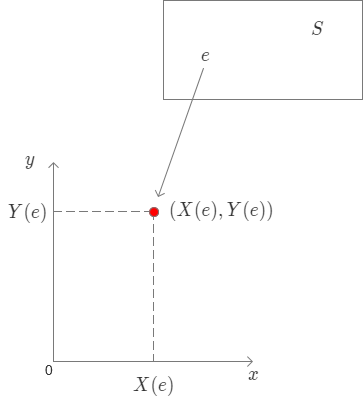二元离散型随机变量

定义： 若二元随机变量 $(X,Y)$ 全部可能取到的不同值是有限时或可列无线对，则称 $(X,Y)$ 是二元离散型随机变量。
（一）离散型随机变量的联合概率分布律
设 $(X,Y)$ 所有可能取值为 $(x_i,y_j)$，称 $P(X=x_i,Y=y_j)=P_{ij}，i,j=1,2,\cdots$ 为二元离散型随机变量 $(X,Y)$ 的联合概率分布律。也可简称 $(X,Y)$ 的分布律。可以用如下图的表格来表示
$\begin{array}{c|ccccc} _X\bcancel{\quad^Y} &y_1&y_2&\cdots&y_j&\cdots \\ \hline x_1 &p_{11}&p_{12}&\cdots&p_{1j}&\cdots \\ x_2 &p_{21}&p_{22}&\cdots&p_{2j}&\cdots \\ \vdots &\cdots&\quad&\cdots&\quad&\cdots \\ x_i &p_{i1}&p_{i2}&\cdots&p_{ij}& \cdots \\ \vdots &\cdots&\quad&\cdots&\quad&\cdots \end{array}$
联合分布律的性质

$p_{ij}\geq 0,$
$\sum_{i=1}^{\infty}\sum_{j=1}^{\infty}p_{ij}=1$
$P((X,Y)\in D)=\sum_{(x_i,y_j)\in D}p_{ij}$

其中 $p_{ij}=P(X=x_j,Y=y_j),i,j=1,2,\cdots$例 1： 一盒子中有 10 件产品，其中 6 件正品 ，4 件次品。从中取 1 件产品检验，不放回，再取 1 件检验。引入如下的随机变量 $X$ 与 $Y$，
$X=\begin{cases} 0, &\text{第 1 次取到次品} \\ 1, &\text{第 1 次取到正品}， \end{cases} \quad Y=\begin{cases} 0, &\text{第2次取到次品} \\ 1, &\text{第2次取到正品}, \end{cases}$
求 $(X,Y)$ 的联合分布律。
解： $(X，Y)$ 可能的取值数对有：$(0,0),(0,1),(1,0),(1,1).$
由乘法公式 $P(AB)=P(A)P(B|A)$ 得：
$P(X=0,Y=0)=P(X=0)P(Y=0|X=0)=\cfrac{4}{10}\times\cfrac{3}{9}=\cfrac{2}{15}$
同理得：$P(X=0,Y=1)=\cfrac{4}{10}\times\cfrac{6}{9}=\cfrac{4}{15}$
$P(X=1,Y=0)=\cfrac{6}{10}\times\cfrac{4}{9}=\cfrac{4}{15},P(X=1,Y=1)=\cfrac{6}{10}\times\cfrac{5}{9}=\cfrac{5}{15}$
$\begin{array}{c|cc} _X\bcancel{\quad^Y} & 0 & 1 \\ \hline 0 & \cfrac{2}{15} & \cfrac{4}{15} \\ 1 & \cfrac{4}{15} & \cfrac{5}{15} \end{array}$

例 2： 设随机变量 $X$ 在 1、2、3、4 四个正数中等可能地取一个值，另外一个随机变量 $Y$ 在 $1\sim X$ 中等可能地取一整数值，试求 $(X,Y)$ 的联合概率分布及 $X、Y$ 的分布。
解： $X、Y$ 的取值情况均为 1,2,3,4；当 $i,j=1,\cdots,4$ 时
$P(X=i,Y=j)=P(X=i)P(Y=j|X=i)=\begin{cases} \cfrac{1}{4}\times\cfrac{1}{i}, &i\geq j \\ \\ \cfrac{1}{4} \times 0, &i
联合概率分布律如下：
$\begin{array}{c|cc} _X\bcancel{\quad^Y} & 1 & 2 & 3 & 4 \\ \hline 1 & \cfrac{1}{4} & 0 & 0 & 0 \\ \\ 2 & \cfrac{1}{8} & \cfrac{1}{8} & 0 & 0 \\ \\ 3 & \cfrac{1}{12} & \cfrac{1}{12} & \cfrac{1}{12} & 0 \\ \\ 4 & \cfrac{1}{16} & \cfrac{1}{16} & \cfrac{1}{16} & \cfrac{1}{16} \end{array}$
求 $X、Y$ 分布律
$P(X=i)=1/4, i=1,2,3,4.$
$事件 \{X=1\},\cdots,\{X=4\}$ 是 $\{Y=j\}$ 前导事件组，由全概率公式得：
$P(Y=j)=\sum_{i=1}^{4}P(X=i)P(Y=j|X=i),j=1,2,3,4.$
所以，$X、Y$ 分布律就是在联合分布律表中横向、纵向相加！

例 3： 袋中有 1 个红球， 2 个黑球，3 个白球，现有放回地取两次，每次取一球，以 $X,Y,Z$ 分别表示两次取球所得的红、黑、白球个数。求：
（1）$P(X=1|Z=0)$
（2）$P(X=1,Z=0)$
（3）$(X,Y)$ 概率分布。
解：
（1） 这一问表示的意思是取到不是白球的前提下，取到 1 个红球的概率，所以：
$\quad P(X=1|Z=0)=\cfrac{1}{3}\times\cfrac{2}{3}+\cfrac{2}{3}\times\cfrac{1}{3}=\cfrac{4}{9}$
（2）这一问表达的是取出 1 个红球跟 0 个白球的概率，所以：
$\quad P(X=1,Z=0)=\cfrac{1}{6}\times\cfrac{2}{6}+\cfrac{2}{6}\times\cfrac{1}{6}=\cfrac{1}{9}$
这里需要注意两问的区别!
（3）$X，Y$ 的取值范围均为 0, 1, 2.
$P(X=0,Y=0)=\cfrac{3}{6}\times\cfrac{3}{6}=\cfrac{1}{4}\quad\quad$ 2 球均为白球
$P(X=0,Y=1)=\cfrac{2}{6}\times\cfrac{3}{6}\times2=\cfrac{1}{3}\quad\quad$ 黑白或者白黑
$P(X=1,Y=2)=0\quad\quad$ 这里总数超过 2 个，不符合条件。
$P(X=2,Y=0)=\cfrac{1}{6}\times\cfrac{1}{6}=\cfrac{1}{36}\quad\quad$ 两球均为红球
其余情况类似可得！
所以 $(X,Y)$ 的概率分布为：
$\begin{array}{c|cc} _X\bcancel{\quad^Y} & 0 & 1 & 2 \\ \hline 0 & \cfrac{1}{4} & \cfrac{1}{3} & \cfrac{1}{9} \\ \\ 1 & \cfrac{1}{6} & \cfrac{1}{9} & 0 \\ \\ 2 & \cfrac{1}{36} & 0 & 0 \\ \\ \end{array}$


展开全文二元随机变量
• 1 随机变量 2 离散型随机变量及其分布律 3 随机变量的分布函数 4 连续型随机变量及其概率密度 5 随机变量的函数的分布人工智能 机器学习 数学
• 设已知离散型随机变量XXX的分布律P(X=xk)=pkP(X=x_k)=p_kP(X=xk​)=pk​，k=1,2,⋯k=1,2,\cdotsk=1,2,⋯及函数Y=g(X)Y=g(X)Y=g(X)为计算YYY的分布律，先算得序列{g(x1),g(x2),⋯ }\{g(x_1), g(x_2), \cdots\}{g(x1...
• 2.2.2.离散型随机变量分布律 设离散型随机变量XXX所有可能取的值为xk(k=1,2,...)x_k(k=1,2,...)xk​(k=1,2,...)，XXX取各个可能值的概率，即事件{X=xkX=x_kX=xk​}的概率，为P(X=xk)=pk,k=1,2,...P(X=x_k)=p_k,k=....
• 做实验计算联合熵需要使用概率密度。 函数accumarray不是太行，方阵中很多的0浪费内存，我可没有几百G的内存ε=(´ο｀*)))唉。 Matlab代码实现 function tong1joint = calmi(u1, u2, wind_size) x = [u1, u2]; //%...算法 matlab
• 1. 二维离散型随机变量的条件分布 2. 二维连续型随机变量的条件分布
• 注：上一小节对随机变量做了一个概述，这一节主要记录一维离散型随机变量以及关于它们的一些性质。对于概率论与数理统计方面的计算及可视化，主要的Python包有scipy, numpy和matplotlib等。 以下所有Python代码...
• 离散型变量分布概率质量函数（分布律），记为pmf。除此之外，每个分布都有一个服从该分部变量发生器函数rvs，用来产生服从该分布的随机数。 1. bernoulli分布（0-1分布） Python的scipy.stats包中，bernoulli类...
• 将一颗骰子抛掷两次，以XXX表示两次中得到的小的点数，试求XXX的分布律. 思路 以Y1,Y2Y_1,Y_2Y1​,Y2​表示第一次、第次投掷时骰子出现的点数，样本空间就可以表示为S={(y1,y2)∣y1=1,2,...,6;y2=1,2,...6}S=\{(y_...
• 需要注意的是：连续型随机变量的模型中的函数值不是在这点的概率，在这点的概率为0，因为随机事件有无数个，平均到这个事件的概率最准确的说法就是0，这点的函数值是概率的密度，就像物质一样，在某个地方的密度越大...
• 概率论对于学习 NLP 方向的人，重要性不言而喻。于是我打算从概率论基础篇开始复习，也顺便巩固巩固基础。 这是基础篇的第四篇知识点总结 知识点：二维离散型随机变量 ...NLP基础
• 文章目录3.1 二维随机变量二维随机变量及其分布函数二维离散型随机变量二维连续型随机变量 二维随机变量及其分布函数 上一章里我们都是用一个随机变量XXX来表述概率的。但是在现实生活里影响事情发生概率的随机变量...
• 借鉴大佬的 下面附上网址 ...随机变量 随机变量是指变量的值无法预先确定仅以一定的可能性(概率)取值的量。 它是由于随机而获得的非确定值，是概率中的一个...离散型随机变量 定义 分布函数性质 个人觉得十分棒的图（思
• 文章目录一、二维离散型随机变量函数的分布二、二维连续型随机变量函数的分布分布密度 一、二维离散型随机变量函数的分布 二、二维连续型随机变量函数的分布分布密度 首先，我们有两个随机变量 X, Y。它们的概率...
• 随机变量与多维随机变量1.随机变量及其概率分布1....二维离散型随机变量的分布3. 二维连续性随机变量的密度4.常见二维随机变量的联合分布5.随机变量的独立性和相关性6.两个随机变量简单函数的概...
• 二维离散型随机变量分布及独立性3. 二维连续型随机变量的分布及独立性4. 二维标准正态分布联合概率密度5. 两个随机变量的函数分布6. 考点分布 1. 多维随机变量及其分布 2. 二维离散型随机变量分布及独...
• 离散型随机变量分布离散型随机变量的定义若随机变量 XX 的可能取值为有限个或可列个，称 XX 为离散型随机变量离散型随机变量分布率 称 P{X=xi}=pi,(i=1,2,⋯) P\{X = x_i\} = p_{i, (i = 1, 2, \cdots)}...
• 本文主要回顾复习了有关一维离散型、连续型随机变量及分布，以及相关性质。这一部分主要以选择题和填空题的形式出现...离散型随机变量X的概率分布离散型随机变量概率分布离散型随机变量X概率分布的性质及分布函...
• 首先根据二维随机变量均匀分布可以直接得到联合概率密度函数，然后再根据公式可以得到X和Y的边缘密度函数，公式一会用图贴出来，但是此时问题来了，为什么X的密度函数是对dy求积分还有积分的上下限怎么确定？...
• 文章目录集合表示法一维离散型随机变量一维连续型随机变量 集合表示法 一维离散型随机变量 一维连续型随机变量
• 1. 随机变量的概念 顾名思义，随机变量就是“其值随机会而定”的变量。随机变量的反面是“确定性变量”，即其值遵循...根据随机变量其可能取的值的全体的性质，可以把随机变量分为2大类，一类是离散型随机变量，比......